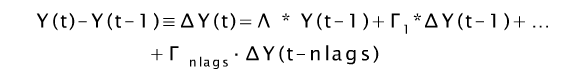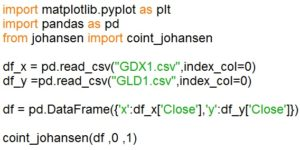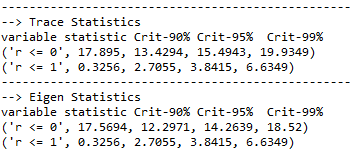# Johansen Cointegration Test: Learn How to Implement it in Python

In this blog post, you will understand the essence of the Johansen Test for cointegration and learn how to implement it in Python. Another popular test for cointegration is the Augmented Dickey-Fuller (ADF) test. ADF test has limitations which are overcome by using the Johansen test.

The ADF test enables one to test for cointegration between two-time series. The Johansen Test can be used to check for cointegration between a maximum of 12-time series. This implies that a stationary linear combination of assets can be created using more than two-time series, which could then be traded using mean-reverting strategies like Pairs Trading, Triplets Trading, Index Arbitrage and Long-Short Portfolio. To learn more about these strategies enroll for the course Mean Reverting Strategies in Python by Dr. E P Chan.Secondly, the ADF test gives different results on changing the order of the two-time series. This is overcome by using the Johansen Test because it is order independent. Let us now look at the mathematics behind the Johansen Test.

### Math behind the Johansen Test

The Johansen test is based on time series analysis. The ADF test is based on an autoregressive model, a value from a time series is regressed on previous values from the same time series. When there are more than one variables, you can still write the relationship of the current prices as a linear function of the past prices in an autoregressive model, but to be more precise this model is then called the Vector Error Correction Model (VECM). Given below is the equation for VECM.In this equation, we have multidimensional variables and hence the multiplication will be matrix multiplication. The coefficients for each of the lag terms in this equation are therefore vector terms.

In the Johansen test, we check whether lambda has a zero eigenvalue. When all the eigenvalues are zero, that would mean that the series are not cointegrated, whereas when some of the eigenvalues contain negative values, it would imply that a linear combination of the time series can be created, which would result in stationarity.

The linear combination of these prices represents the net market value of the portfolio. If the change in the value of the portfolio is related to its current value by a negative regression coefficient or in this case a negative eigenvalue, then we would have a mean reverting or stationary portfolio. This is the essence of the Johansen Test.

### Python implementation of Johansen Test

Let us now implement the Johansen Test in Python on a pair of assets, here we have taken the GLD - GDX pair as an example, GLD is the SPDR Gold Trust ETF and GDX is the Gold Miners ETF. We can expect both of these assets to be correlated, we will now check whether these assets are cointegrated if so we could then create a pairs trading strategy on this pair which will prove to be profitable. Go through the code mentioned below:We will start by importing two libraries. The first library to be imported is the Pandas library which will be used to read data from a CSV file and then to create a data frame containing data of the two instruments.

Secondly, we will be importing the coint_johansen function from the Johansen library, which is a function developed by James LeSage at the Department of Economics, University of Toledo. You can download this code from here.

Once the libraries have been imported, we store the data for the two securities in the variables dfx and dfy by reading data from csv files. Next, we create a data frame df which stores the two-time series for which we have to run the Johansen test.

We then call the coint_johansen function by passing the data frame storing the time series data (df), 0 and 1 as its three arguments. The second term in the arguments represents the order of null hypothesis, a value of 0 implies that it is a constant term, there is no time trend in the polynomial. The third term specifies the number of lagged difference terms used when computing the estimator, here we use a single lagged difference term.

The output of this test provides us with trace statistics and eigen statistics.The trace statistics tell us whether the sum of the eigenvalues is 0. The null hypothesis, r<=0 gives us a trace statistic of 17.895, hence the null hypothesis can be rejected at a 95% confidence level, as the magnitude of the trace statistic is greater than the critical value, note that the Johansen test only gives the magnitude of the output, hence we need not worry about the signs.

The eigen statistics stores the eigenvalues in decreasing order of magnitude, they tell us how strongly cointegrated the series are or how strong is the tendency to mean revert. In our example, the eigen statistic for the null hypothesis can be rejected at a 95% confidence level, because 17.5694 is greater than 14.2639.

The eigenvectors give us the equation of the mean-reverting linear combination of the time series. The eigenvector corresponding to the highest eigenvalue represents the portfolio which has the greatest mean-reverting property. The null hypothesis was that the time series are not cointegrated, hence when we reject the null hypothesis and accept the alternate hypothesis, we suggest that the series are cointegrated.

### Properties of Johansen Test

The Johansen test will give the same result even if the order of the time series is reversed, you can try this as an exercise. This test can be used as an order independent way to check for cointegration. This test allows us to check for cointegration between triplets, quadruplets and so on up to 12-time series.

The reason is simply that no mathematician was able to compute the critical values for more than 12 variables. Hence the result cannot be used to reject the null hypothesis. The vector error correction model can be used on even 1000 stocks depending on the availability of computing power. It would not be able to tell whether the stocks are cointegrating but it can still be used as a prediction model.

### Next Steps

Learn to optimize your portfolio in Python using Monte Carlo Simulation. This article explains how to assign random weights to your stocks and calculate annual returns along with standard deviation of your portfolio that will allow you to select a portfolio with maximum Sharpe ratio.